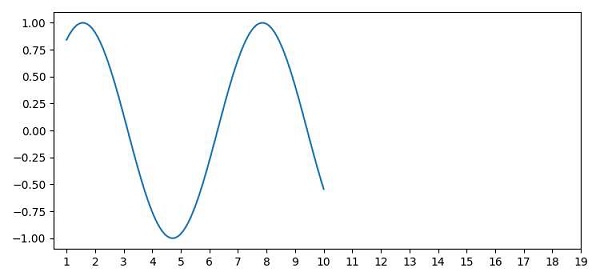# Adding extra axis ticks using Matplotlib

To add extra ticks in matplotlib, we can take the following Steps −

• Create x and y points using numpy.

• Plot x and y points over the plot, where x ticks could be from 1 to 10 (100 data points) on the curve.

• To add extra ticks, use xticks() method and increase the range of ticks to 1 to 20 from 1 to 10.

• To display the figure, use the show() method.

## Example

import numpy as np
from matplotlib import pyplot as plt
plt.rcParams["figure.figsize"] = [7.50, 3.50]
plt.rcParams["figure.autolayout"] = True
x = np.linspace(1, 10, 100)
y = np.sin(x)
plt.plot(x, y)
plt.xticks(range(1, 20))
plt.show()

## Output